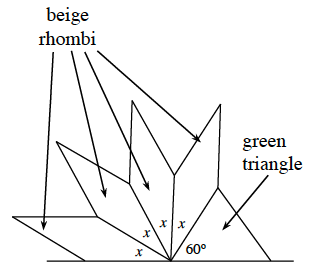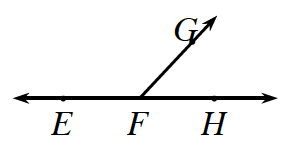### Home > CCAA > Chapter 8 Unit 7 > Lesson CC2: 8.3.2 > Problem8-74

8-74.

Alexander and Santiago put five pattern blocks together with one vertex of each block touching, as shown in the diagram at right. Find the measure of the smaller angle of a beige rhombus.Look at the information below from the Math Box in Lesson 8.3.2.
How are the angles in the diagram similar to the angles in the example?

If two angles have measures that add up to $180°$, they are called supplementary angles. For example, in the diagram at right, $\angle EFG$ and $\angle GFH$ are supplementary because together they form a straight angle.Because all the angles add up to $180°$, you can set up the equation:
$x+x+x+x+60°=180°$

Combine like terms:
$4x+60°=180°$

Solve for $x$.

$x=30°$